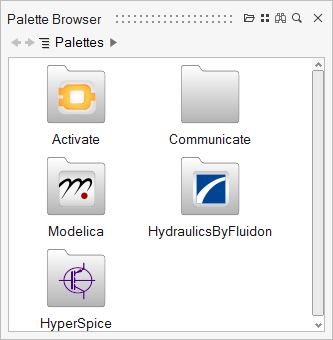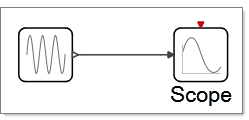# Diagrams

A diagram is an assembly of blocks, links, annotations and other components inside of a model.

Many diagrams can be associated with a single model in which one diagram is defined as the main diagram of a hierarchical structure. The following is the main diagram of a model named Watertank:
Additional diagrams can be present inside of a main diagram if the main diagram includes one or more super blocks. The main diagram of the model, Watertank, includes two super blocks, Controller and Tank, each of which contains a diagram. Double-clicking a super block exposes its internal diagram. The following figure shows the diagram of the Controller super block:
The default setting for the tree in the Project Browser displays a branch for the main diagram of a model and a branch for each diagram associated with a super block. For the model, Watertank, the Project Browser displays a branch for the main diagram, Watertank, and the super blocks Controller and Tank. To see all of the blocks associated with a model, including the basic blocks, you can modify the filter of the Project Browser to include all blocks. The following figure shows all of the blocks that belong to the Controller super block:

## Creating Diagrams

The process to create a diagram includes adding, linking, and assembling blocks in the modeling window. The blocks in a diagram can include predefined blocks from the library palettes or user-defined blocks.

## Adding Library Blocks to a Diagram

Add a library block to a diagram from the Palette Browser.

1. To open the Palette Browser, press F6 or select View > Palette Browser.The Palette Browser displays the installed library palettes.
2. Select a library, then select a palette.
The Palette Browser displays the blocks available in the selected palette.
3. Select a block that you want to add to your diagram, then drag and drop it into the modeling window.
The following example shows three blocks from the Activate library that have been added to a diagram:
• Activate > SignalGenerators > SineWaveGenerator
• Activate > SignalViewers > Scope
• Activate > ActivationOperations > Eventclock

## Adding User-Defined Blocks to a Diagram

Add or import a user-defined or customized block that is not associated with the installed libraries into a diagram. These blocks are stored as .scb files.

1. Select File > Libraries > Insert block from library.
2. From the File Browser that appears, select the .scb file you want to include in your diagram.
The file you specify appears as a block inside of the diagram.

## Copying Blocks to a Diagram

From inside of a diagram, you can copy a block and then paste it into the current model or into any open model.

1. In a diagram, select a block you want to copy, right-click, and from the context menu, select Copy.
2. Select a diagram in the current model or any open model, right-click and from the context menu, select Paste.

## Duplicating Blocks in a Diagram

The Duplicate option lets you easily add multiples of a block into a diagram.

1. From a diagram, select a block you want to duplicate, and right-click.
2. From the context menu, select Duplicate.
An exact duplicate of the block appears in the diagram.

## Linking Blocks in a Diagram

Connect blocks with links that extend between the ports on the blocks.

1. Locate two blocks in a diagram that you want to connect.
2. Click the output port of the first block, then click the input port of the second block.

A link appears between the blocks and connects the blocks together. The link includes a snapping feature that keeps the link segments straight and makes picking ports easier when connecting blocks.

Note: Certain blocks require that External Activation ports be turned on before the blocks can accept a link.
The following image shows you a SineWaveGenerator block and a Scope block connected with a link:## Diagram Parameters

Diagram parameters are variables that can be used for defining block parameters.

### Overview of Diagram Parameters

Most blocks contain parameters with user-defined values. Block parameter values can be any OML valid expression. Such an expression may be a simple number, for example 12; an expression involving numbers and strings, such as sin(cos(3)-1); or even an expression involving OML variables such as exp(a^2). In the latter case, the variable a is a diagram parameter.

Diagram parameters are OML variables that are associated with a diagram. They can be used in the definition of block parameter values for blocks within the diagram. The set of these variables and their values constitute an OML workspace.

The parameters of a diagram D are defined by the context of the diagram D, the contexts of the diagrams that include D, and the model Initialization script. For example, let D0, D1, … Dn represent the parent diagrams of D with Dn being the closest and D0 the furthest parent. In this case, the scoping rule in the software is such that the value of a diagram parameter is given by the definition of the corresponding variable if the variable is defined in the context of D. If the variable is not defined, the definition of the closest parent diagram provides the value of the parameter.

In the absence of such a definition, the definition in the Initialization script of the model provides the value of the parameter. More specifically, the workspace of Diagram D, which is the set of diagram D parameters, is obtained as follows:

• The Initialization script of the model is evaluated resulting in a workspace, the elements of which are called model parameters.
• The context of D0 is evaluated in this workspace resulting in the workspace of Diagram D0.
• The context of D1 is evaluated in the workspace of Diagram D0, yielding the workspace of Diagram D1, and so on.
• Finally the workspace of Diagram D is obtained by evaluating its context in the workspace of its parent, Diagram Dn.
Note: The evaluations of the diagram and block parameters are done at run time. Therefore, if the Initialization script or a diagram context contains instructions to load data or run an external script defining new variables, the values of these variables may change if the external files are modified from the time of one simulation run to another. If the expression corresponding to a block parameter value refers to a variable that is not a diagram parameter, an error occurs at run time.